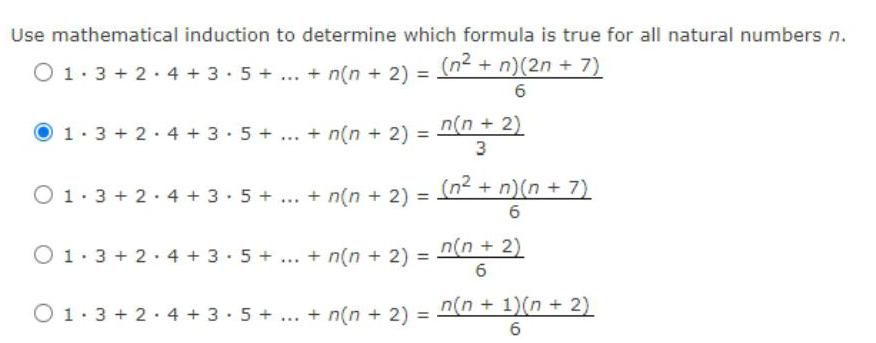Question:

# Use mathematical induction to determine which formula is

Last updated: 7/4/2022Use mathematical induction to determine which formula is true for all natural numbers n. 01.3 +2.4+3.5 + ... + n(n + 2) = (n² + n)(2n + 7) 6 1 3+2 4+3.5+ O1 3+2 4+3 5+ *** *w* + n(n + 2) = + n(n + 2) = O1 3+2 4+3 5+ ... + n(n+ 2) = n(n + 2) 3 (n² + n)(n+7) 6 n(n + 2) 6 01.3+2 4+3.5+...+ n(n+ 2) = n(n + 1)(n + 2) 6